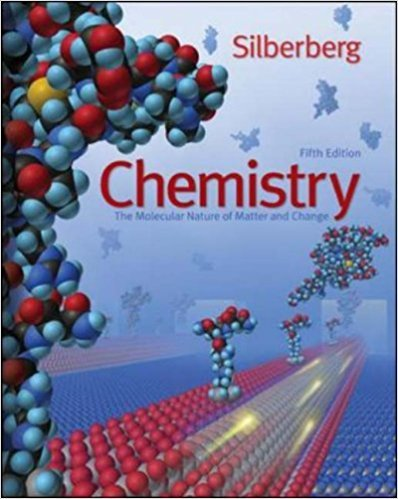×
×

# Consider two 1-L samples of gas: one is H2 and the otherISBN: 9780077216504 103

## Solution for problem 5.67 Chapter 5

Chemistry: The Molecular Nature of Matter and Change | 5th Edition

• Textbook Solutions
• 2901 Step-by-step solutions solved by professors and subject experts
• Get 24/7 help from StudySoup virtual teaching assistantsChemistry: The Molecular Nature of Matter and Change | 5th Edition

4 5 0 334 Reviews
30
3
Problem 5.67

Consider two 1-L samples of gas: one is H2 and the other is O2. Both are at 1 atm and 25 C. How do the samples compare in terms of (a) mass, (b) density, (c) mean free path, (d) average molecular kinetic energy, (e) average molecular speed, and (f) time for a given fraction of molecules to effuse?

Step-by-Step Solution:
Step 1 of 3

· ~ ic.uJJ .t~-o ~. N!eden~- W.-"OS bCY-:\ cC\t.QrnJ~e ~..rdr-o-~_-~i ~_ nuclei betw_e_edi~~ .cL-r.n~ .3 N· ·- - -·. CtllOW · ;l0, ore. bod!L_ ..,uo1Sing~-.:f'i. _P leIi: .cc.it- ~~~ccoo;t1Y) bonOf~· orDrn04_l b1of'l__ce, rf_ v ~ x'f\...

Step 2 of 3

Step 3 of 3

##### ISBN: 9780077216504

The full step-by-step solution to problem: 5.67 from chapter: 5 was answered by , our top Chemistry solution expert on 09/09/17, 04:57AM. The answer to “Consider two 1-L samples of gas: one is H2 and the other is O2. Both are at 1 atm and 25 C. How do the samples compare in terms of (a) mass, (b) density, (c) mean free path, (d) average molecular kinetic energy, (e) average molecular speed, and (f) time for a given fraction of molecules to effuse?” is broken down into a number of easy to follow steps, and 58 words. This textbook survival guide was created for the textbook: Chemistry: The Molecular Nature of Matter and Change, edition: 5. Since the solution to 5.67 from 5 chapter was answered, more than 259 students have viewed the full step-by-step answer. This full solution covers the following key subjects: average, Samples, Molecular, given, consider. This expansive textbook survival guide covers 22 chapters, and 2776 solutions. Chemistry: The Molecular Nature of Matter and Change was written by and is associated to the ISBN: 9780077216504.

Unlock Textbook Solution